# CIE A Level Chemistry复习笔记1.5.2 Energy Level Diagrams

### Energy Level Diagrams

• An energy level diagram is a diagram that shows the energies of the reactants, the transition state(s) and the products of the reaction with time
• The transition state is a stage during the reaction at which chemical bonds are partially broken and formed
• The transition state is very unstable – it cannot be isolated and is higher in energy than the reactants and products
• The activation energy (Ea) is the energy needed to reach the transition state
• We can define the activation energy as ‘the minimum amount of energy needed for reactant molecules to have a successful collision and start the reaction’The energy level diagram for the reaction of hydrogen with chlorine to form hydrogen chloride gas

#### Exothermic reaction

• In an exothermic reaction, the reactants are higher in energy than the products
• The reactants are therefore closer in energy to the transition state
• This means that exothermic reactions have a lower activation energy compared to endothermic reactions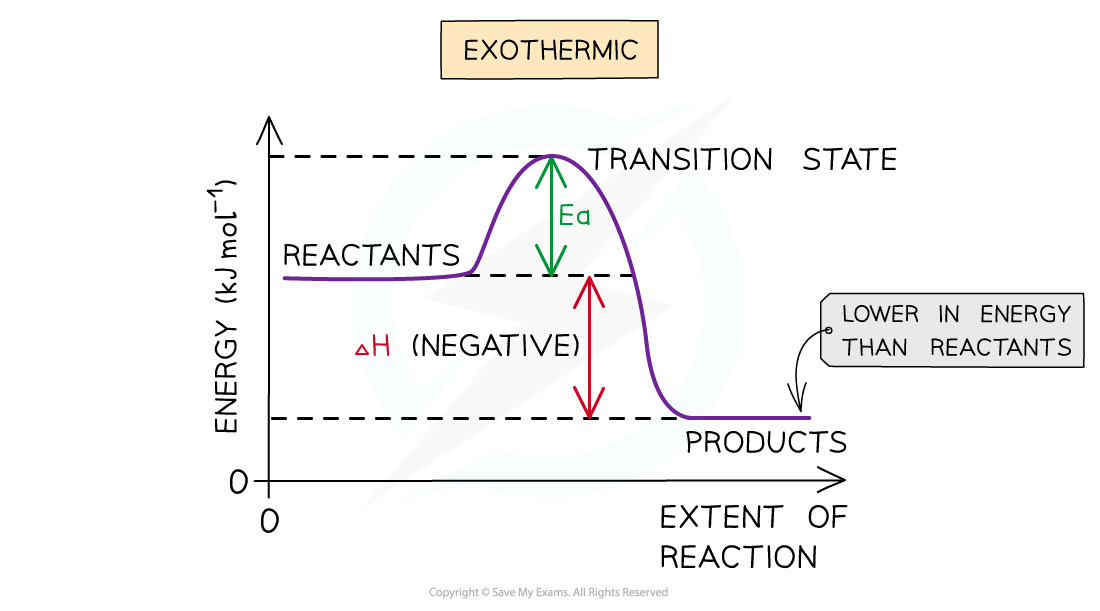The energy level diagram for exothermic reactions

#### Endothermic reaction

• In an endothermic reaction, the reactants are lower in energy than the products
• The reactants are therefore further away in energy to the transition state
• This means that endothermic reactions have a higher activation energy compared to exothermic reactions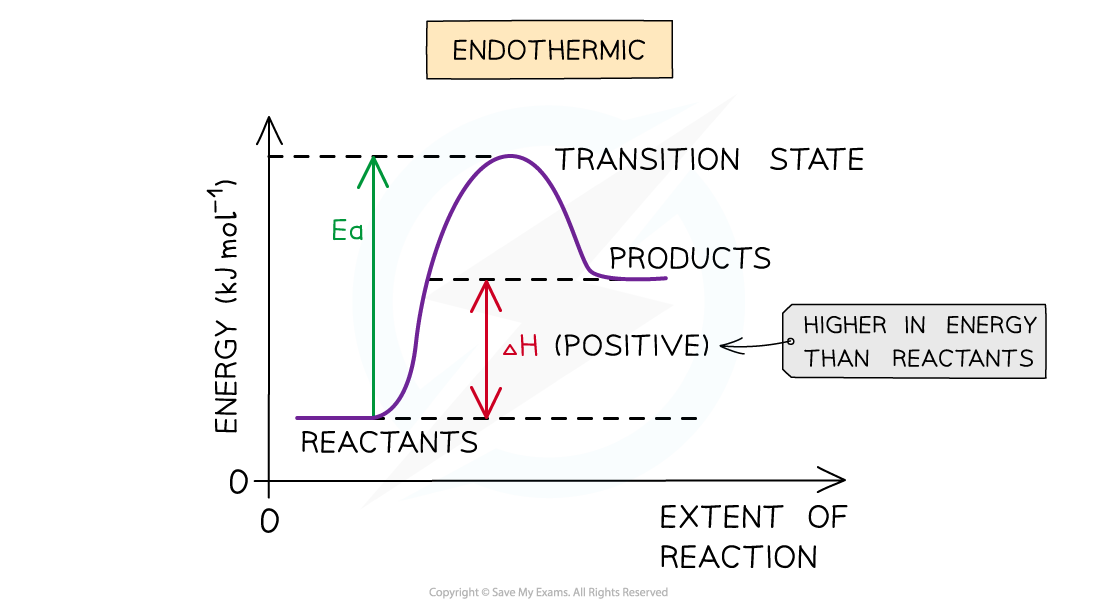The energy level diagram for endothermic reactions

#### Worked example: Drawing energy level diagrams of the combustion of methane• Step 1: The chemical equation for the complete combustion of methane is:

CH(g) + 2O2 (g) → CO2 (g) + 2H2O (l)

• Step 2: Combustion reactions are always exothermic (ΔH is negative) so the reactants should be drawn higher in energy than the products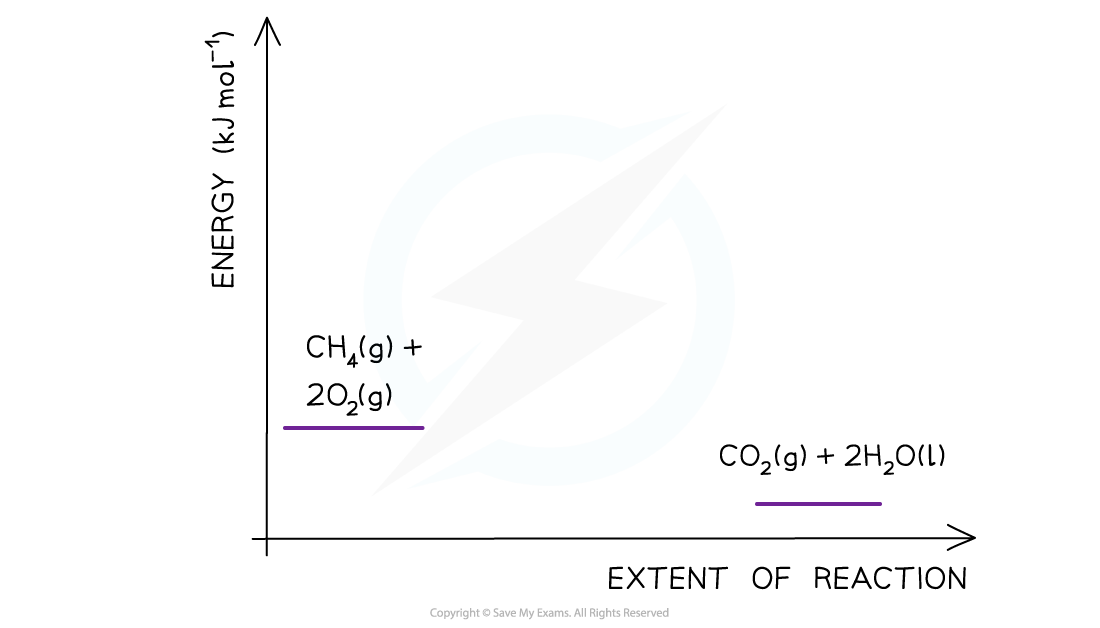• Step 3: Draw the curve in the energy level diagram clearly showing the transition state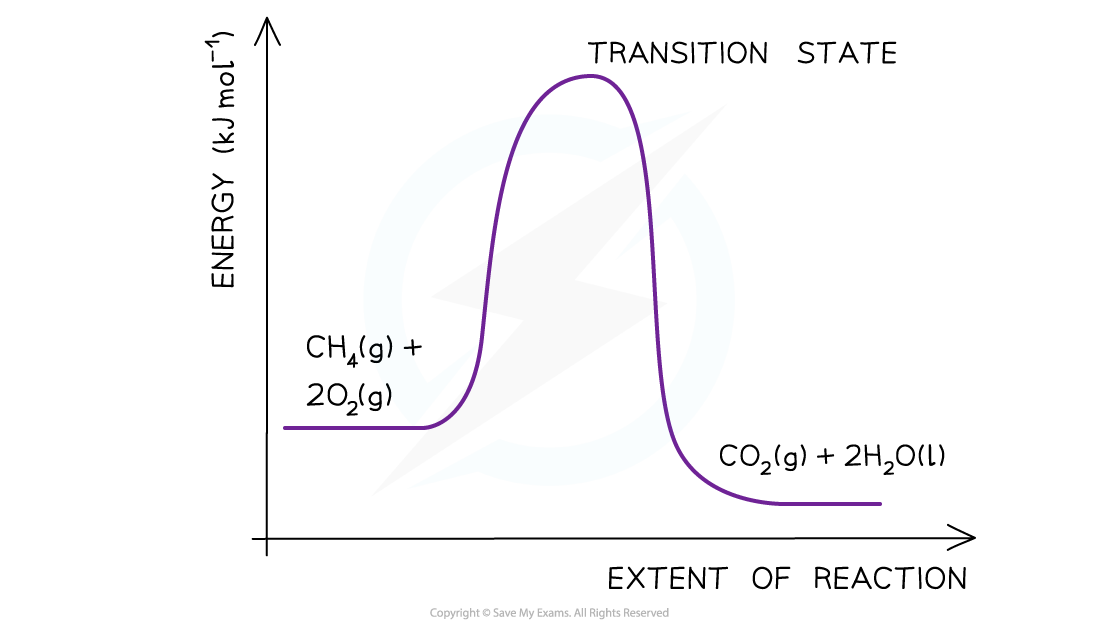• Step 4: Draw arrows to show the Ea and ΔH  including their values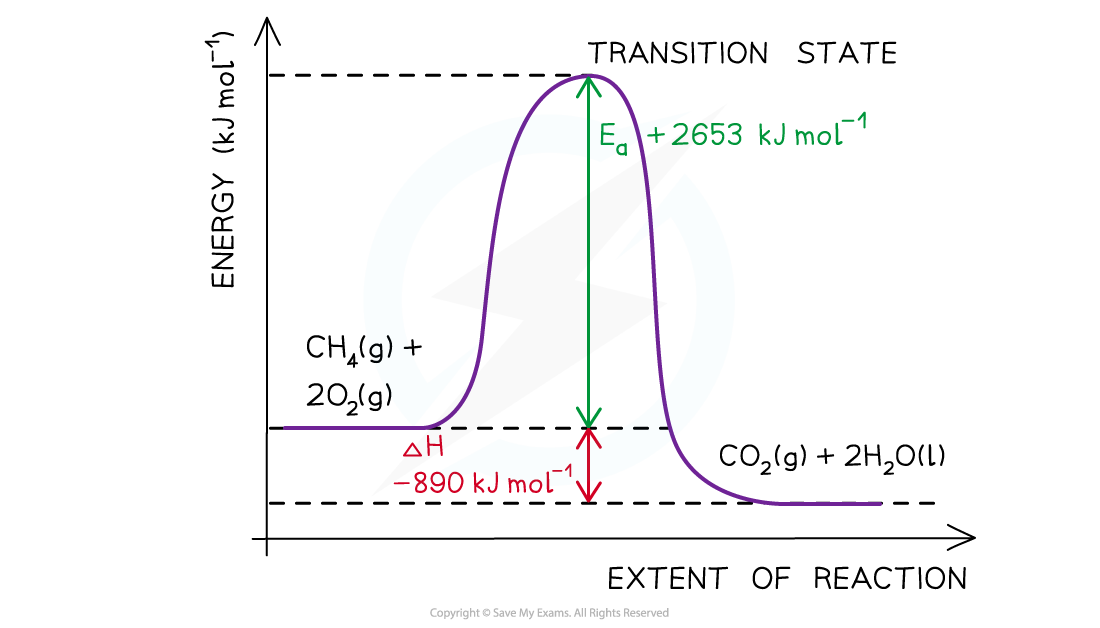#### Worked example: Determining the activation energy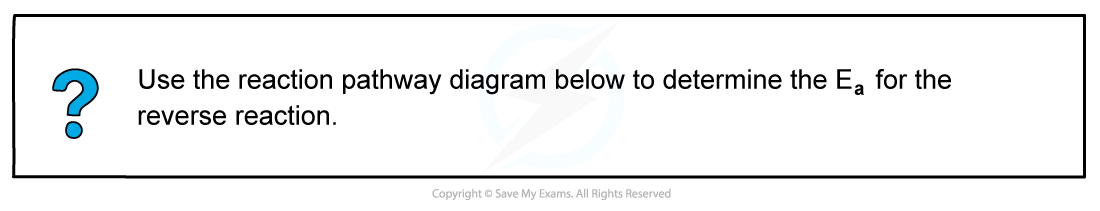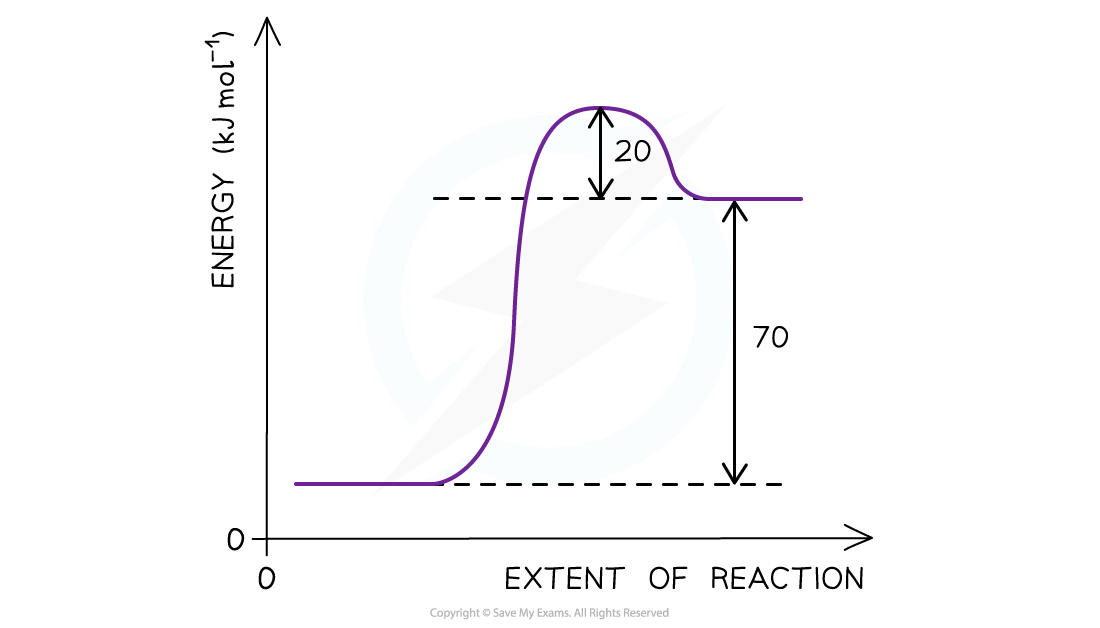The reaction pathway diagram for a reversible reaction

The Ea  is the energy difference from the energy level of the reactants to the top of the ‘hump’

Ea (forward reaction) = (+70 kJ mol-1) + (+ 20 kJ mol-1 ) = +90 kJ mol-1

As the question is asking for the reverse reaction the Ea  is the energy difference from the energy level of the products to the ‘hump’

Ea (reverse reaction) = +20 kJ mol-1

#### Exam Tip

The activation energy is the energy difference from reactants to transition state.The enthalpy change of the reaction is the energy difference from reactants to products.Remember to label the axis of the energy level diagrams!